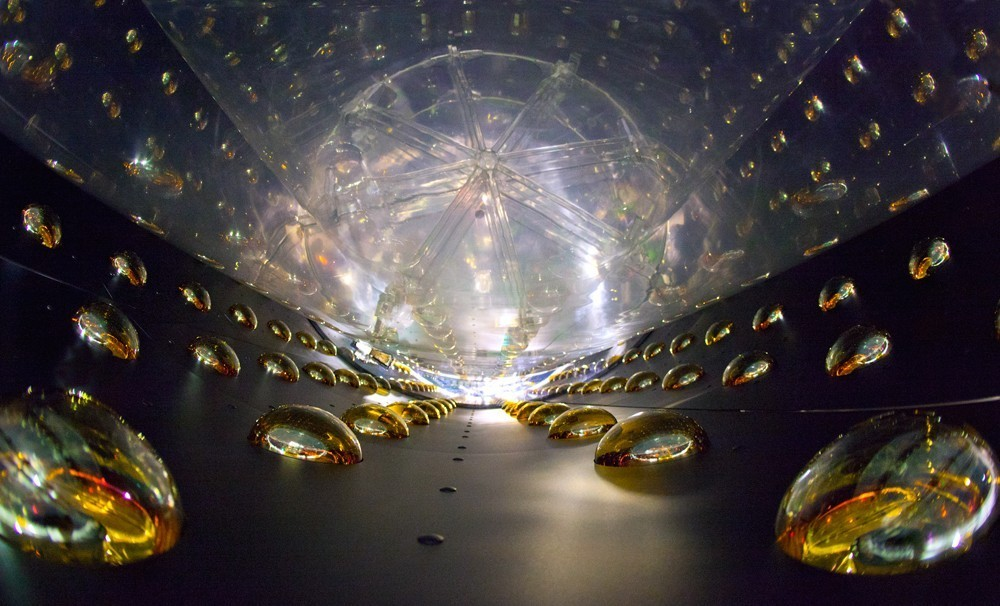# Easing Tension With Neutrinos

In Particle Physics by Brian Koberlein0 Comments

In an earlier post I wrote about the solar neutrino problem, and how it was resolved by recognizing neutrinos can change flavor.  The fact that neutrinos can do this means they cannot be traveling at the speed of light, and so they have mass.  Earlier theories of particle physics had assumed that neutrinos were massless, but we now know this assumption was wrong.  But while we now know the three flavors of neutrinos have mass, we don’t know what their masses are.  Now a new paper published in Physical Review Letters has given us an idea of their mass, and it does so by alleviating a bit of cosmic tension.

Galaxy clusters put an upper limit on neutrino mass.
Credit: Sloan Digital Sky Survey.

Moments after the big bang, the initial electrons, photons and neutrinos of the universe were produced.  This means the number of neutrinos in the universe is related to the number of photons.  We can calculate the photon number by observing the cosmic microwave background, so we have a good idea of the number of neutrinos in the universe.  If the neutrinos had a larger mass their gravity would affect the distribution of galaxies, specifically what is known as the Baryon Acoustic Oscillation, or BAO.  Galaxy surveys see no such effect, which suggests the total mass of all three neutrino types can be no more than 0.23 eV, or less than 2 millionths the mass of an electron.  Observations of neutrino oscillations also require that at least one flavor of neutrino has a mass of at least 0.04 eV.

Different measures of the universe give slightly different values for dark matter and dark energy. Credit: ESO.

The BAO is one way we can determine the structure of the universe, in particular the amount of dark matter and dark energy it has.  There are two other main ways, which are the cosmic microwave background (CMB) which is a measure of the remnant heat of the big bang, and the gravitational lensing of distant galaxies.  Each of these gives slightly different results for the various cosmological parameters that describe the universe.  Based on the cosmic microwave background, we would expect to see slightly less galaxy clustering than we actually observe.  The amount of gravitational lensing we see is less than expected from the two other measures.

Because neutrinos have mass and weakly interact with other particles, they are often brought up as a possible solution to dark matter.  However neutrinos have such a small mass that they cannot be the solution to the dark matter problem.  But neutrinos could ease some of the tension between our different cosmological measures.

In this new paper, the authors looked at how massive neutrinos would affect the cosmological measures we observe.  What they found was that massive neutrinos would work to increase the amount of galaxy clusters we see, and they would decrease the amount of gravitational lensing we observe.  So the presence of neutrinos would bring our different measures into better agreement.  The best agreement between these three measures is found when the total mass of all three neutrino types is  0.320 +/- 0.081 eV.

So it seems the mystery of neutrino mass can solve the observational tension that has been bugging cosmologists.

Paper: Battye RA, Moss A. Evidence for Massive Neutrinos from Cosmic Microwave Background and Lensing Observations. Phys. Rev. Lett. 2014;112(5)